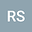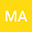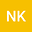loading page

Environmental factors prediction in preterm birth using comparison between logistic regression and decision tree methods: an exploratory analysis
•••Madhu Anand
Dr Bhim Rao Ambedkar University
Author ProfileNeha Kumari
SRM University - Sikkim
Author Profile## Abstract

Objective The main objective of this paper is to compare the performance of logistic regression and decision tree classification methods and to find the significant environment determinants that causes pre-term birth. Design, setting and population Between 2017 to 2018, 90 pregnant females underwent birth outcome followed by research staff at our institutions, out of those 50 are full-term and 40 are preterm births in this study. Method Before and after feature selection logistic regression and decision tree classifier model has been compared in this dataset and to evaluate the model accuracy. Main outcome measures Preforming the accuracy of machine learning classification model and important factors on pre-term birth. Results: Using chi-square test and find the Area of residence and GSH, MDA, α-HCH, total HCH and total DDT are responsible for the preterm birth. Using the multiple logistic regression, pre term birth was associated with MDA and α-HCH (95% CI 0.04 to 0.48 and 95% CI 0.82 to 0.97). The logistic and decision tree model comparison result shows that logistic regression is better in terms of metrics (precision = 0.92, F1-score = 0.96 and AUROC = 0.97), while decision tree performs the poor (precision = 0.75, F1-score = 0.86 and AUROC = 0.87). Conclusions The logistic regression is accurate model to predict the pre-term as compare to decision tree method. The variables like α-HCH , total HCH and MDA (Malondialdehyde) are the most influential factors for preterm birth.